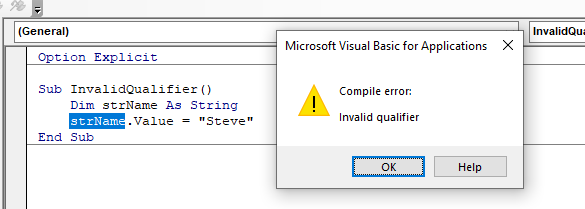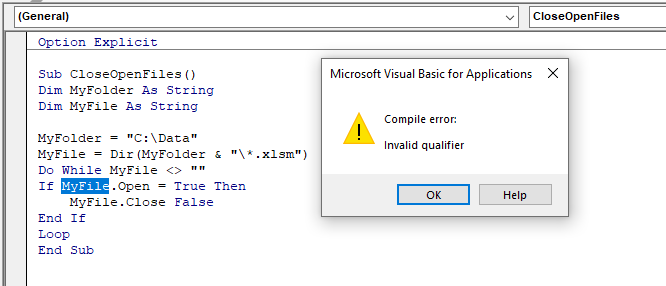# Invalid Qualifier VBA Error

The VBA invalid qualifier error occurs when trying to use properties or methods on a variable or object that do not actually exist for that variable or object.

## String Variables and Properties

A simple example is when trying to assign a value to a string variable.

For example:

``````Sub InvalidQualifier()
Dim strName As String
strName.Value = "Steve"
End Sub``````

When we run this code, we would get the following error:A simple alteration will fix our code:

``````Sub InvalidQualifier()
Dim strName As String
strName= "Steve"
End Sub``````

The value property is not available to a string variable and is not required to populate the variable.

## Object and Methods

Another example could be when trying to work with an Object.

Take the following code for example:In the above code, the variable MyFile has been declared as a String variable. The code is trying to close the file if the file is open – but as a string variable is not an Object, you cannot assign it any properties or methods and therefore you will get this error.

To fix this error, you need to use an Object. We can declare a variable for a workbook and then create a second For Each Loop to check if the workbook name is equivalent to the string variable MyFile. If the workbook name is equivalent to this variable, we can use the Close method of the workbook object to close the file.

``````Sub CloseOpenFiles()
Dim MyFolder As String
Dim MyFile As String
Dim wkb As Workbook

MyFolder = "C:\Data"
MyFile = Dir(MyFolder & "\*.xlsm")
Do While MyFile <> ""
For Each wkb In Workbooks
If wkb.Name = MyFile Then
wkb.Close False
End If
Next wkb
MyFile = Dir
Loop
End Sub``````